# Nonlinear Generalized Companding Transform

3300 words (13 pages) Essay in Computer Science

17/07/18 Computer Science Reference this

Disclaimer: This work has been submitted by a student. This is not an example of the work produced by our Essay Writing Service. You can view samples of our professional work here.

Any opinions, findings, conclusions or recommendations expressed in this material are those of the authors and do not necessarily reflect the views of UK Essays.

### Nonlinear Generalized Companding Transform for Peak-to-Average Power Ratio Reduction in OFDM

• Eashendra Singh

Abstract  One of the main drawback of Orthogonal Frequency Division Multiplexing (OFDM) system is its high Peak-to-Average Power Ratio (PAPR) of the OFDM signal. In this paper a novel non-linear generalized companding scheme called “Quadrilateral Companding Transform (QCT)” has been proposed to reduce the PAPR of OFDM signal. The proposed method provides additional degrees of freedom in comparison to existing trapezoidal companding, exponential companding and trapezium distribution based companding schemes. This allows more flexibility in designing the companding function, which is useful for the overall OFDM system to achieve low BER with good PAPR reduction capability.

Keywords – Complementary cumulative distribution function (CCDF), peak-to-average power ratio (PAPR), orthogonal frequency division multiplexing (OFDM), bit error rate (BER).

## INTRODUCTION

The modern day phenomenon of increased thirst for more information and the explosive growth of new multimedia wireless applications have resulted in an increased demand for technologies that support very high speed transmission rates, mobility and efficiently utilize the available spectrum and network resources. OFDM is one of the best solutions to achieve this goal and it offers a promising choice for future high speed data rate systems , . OFDM has been standardized as part of the IEEE 802.11a and IEEE 802.11g for high bit rate data transmission over wireless LANs . It is incorporated in other applications and standards such as digital audio broadcasting (DAB), digital video broadcasting (DVB), the European HIPERLAN/2 and the Japanese multimedia mobile access communications (MMAC) , . However, a major drawback of FDM systems is the high peak-to-average power ratio (PAPR) of the transmitted signals, resulting in the lower power efficiency, serious signal distortion and out-of-band radiation when the high power amplifier (HPA) is utilized.

Many companding schemes - have been proposed in the literature to reduce the PAPR of OFDM signal. The conventional μ – law and A-law companding schemes can be used for PAPR reduction, by choosing the suitable value of the parameters μ or A, controlling the nonlinearity of the μ -law  or A -law companding function respectively. But the error performance of both the schemes degrades as both of them introduce high companding distortion in OFDM signal at higher values of μ or A. A nonlinear companding transform  has been proposed by Jiang et al. to effectively reduce the PAPR of the OFDM signal. In this scheme , the Gaussian distributed in-phase (I) and quadrature-phase (Q) components of discrete time complex OFDM signal are transformed into a quasi-uniform distribution. In this scheme, the companding function is separately applied to I and Q components of the OFDM signal. The large values of I or Q components of the OFDM signal are compressed, whereas those with small I and Q components are enlarged. The PAPR reduction capability and BER performance of this scheme , can be optimized by properly choosing the parameters of the companding function. Jiang et al. proposed “Exponential Companding (EC)” scheme  to transform Rayleigh distributed OFDM signal magnitude into uniform distribution. Exponential companding has the advantage of maintaining the constant average power level in the nonlinear companding operation. However, the distribution of large signals is increased by the uniform companding, which makes the PAPR reduction was very limited under the bit error rate (BER) performance degradation. In this paper proposed technique transform the Rayleigh distributed OFDM signal magnitude into Quadrilateral distribution function as shown in figure 2 to achieve an additional degree of freedom over TC . The parameters of quadrilateral distribution are chosen in such a way that it produces least possible companding distortion to achieve low BER for a given PAPR.

The remainder of this paper is organized as follows: In section II, the OFDM system model with quadrilateral companding. The proposed quadrilateral companding and decompanding functions are derived in section III. Mathematical analysis of the PAPR performance of proposed scheme is presented in section IV, simulation results for PAPR performances of the proposed scheme are presented and discussed in the same section and conclusion is summarized in section V.

## SYSTEM MODEL

The block diagram of an OFDM system using companding scheme for PAPR reduction is shown in Fig. 1. Here, I have considered an OFDM system with N subcarriers, in which each of the subcarrier is each of the subcarrier is modulated by M-PSK or M-QAM. As shown in Figure 1.The input binary data sequence is first converted into N parallel data substreams and then these are mapped to the constellation points of M- PSK or M-QAM to achieve desired modulation on each of the subcarriers. After this, subcarrier modulation is performed using IFFT block to obtain the discrete time domain OFDM signal. Let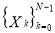be the N complex modulated data symbols to be transmitted over N subcarriers. The discrete time domain OFDM signal generated after taking IFFT of a block of N modulated data symbols. Discrete time domain OFDM signal is passed through the parallel to serial (S/P) converter and then applied to the compander for reducing the dynamic range or PAPR of the OFDM signal. The companded OFDM signal is applied to digital to analog (D/A) converter to get analog signal and then finally amplified using HPA. At the receiver, the received signal is first converted into digital signal using A/D converter.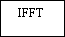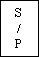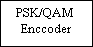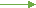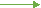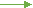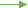Data in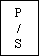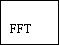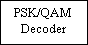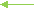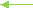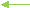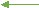Data out

Figure 1. Block diagram of OFDM with companding

The digital signal is then expanded by inverse companding function known as decomapnding function. After that subcarrier demodulation is performed by taking the FFT of OFDM signal obtained from expander. Finally, M-PSK or M-QAM decoder is used to decode the received data signal.

## PROPOSED COMPANDING TECHNIQUE

The quadrilateral companding function h(x) is a nonlinear companding function. It transforms the original probability distribution function of OFDM signal magnitude into a quadrilateral distribution as shown in Figure 2, and hence the name “Quadrilateral Companding Transform”.This may also be called nonlinear generalized companding transform.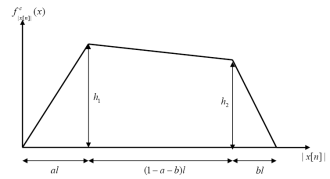Figure 2. Quadrilateral distribution for proposed QCT

The symbols notation used throughout this paper are listed in Table 1 for convenience.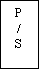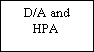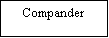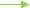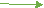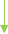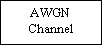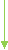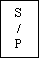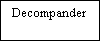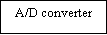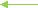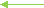Table 1: List of symbols used in QCT

 kth modulated data symbol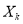nth sample of discrete time domain OFDM Signal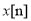PDF of original OFDM signal (without companding)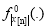CDF of original OFDM signal (without companding)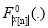PDF of OFDM signal after companding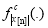CDF of OFDM signal after companding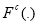Upper-bound of the peak value of OFDM signal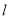Quadrilateral Companding function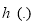Quadrilateral Decompanding function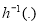The pdf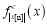of quadrilateral trapezium distribution can be read from Figure 2 aswhere h1 , h2, l, a and b are the parameters of quadrilateral distribution as shown in the Figure 2.These parameters (h1 , h2, l, a and b) control the nonlinearity of the companding functions. The cumulative distribution function (CDF) of quadrilateral distribution function can be calculated using the following relationship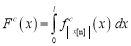(2)

Using (1) and (2) we haveQuadrilateral distribution function is bounded in the interval [0,l]. Like EC, TC and TDBC, in this scheme also average power of the OFDM signal before and after companding is kept same, therefore we have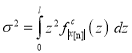(3)As shown in Figure 2, the PDF of quadrilateral trapezium companded OFDM signal lies in the interval [0,l] , therefore, we have,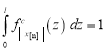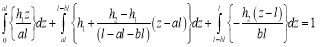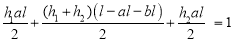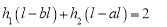(4)

For given values of l, a and b, the parameters ( h1 , h2 ) of the companding function h(x) can be easily calculated using (3) and (4). Therefore, three parameters (l, a and b ) can be chosen independently to control the nonlinearity of companding function h(x) . Hence the proposed QCT has three degree of freedoms. The values of l, a and b should be chosen independently to provide low PAPR and BER.

The expression of QCT function h(x) can be derived after equating the CDF of original and companded OFDM signal. Therefore, we have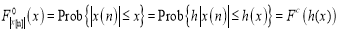Where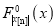is the CDF of original OFDM signal given by following: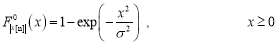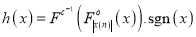(5)

Therefore we haveThe output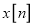of the N-point Inverse Fast Fourier Transform (IFFT) ofare the OFDM signal sample over one symbol interval, or mathematically,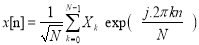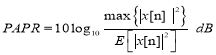Where E [.] denotes the expectation operator.

## PERFORMANCE ANALYSIS

In , the PAPR and BER performance of TC has been evaluated for (a = 0.4,b = 0.1 and l = 1.633) , (a = 0.2,b = 0.7 and l = 2.164) , (a = 0,b = 0 and l = 1.732) , (a = 0.9, b = 0.1 and l = 1.488) and (a = 0,b = 1 and l = 2.449) , here we refer to them as ‘TC-1’, ‘TC-2’, ‘EC’, ‘TC-3’ and ‘TC-4’ respectively. In , it has been shown that TC-3 provides the best PAPR reduction capability among all the cases under consideration, but its BER performance is very poor, on the other extreme TC-4 provides very less PAPR reduction. Therefore, we ignore these two cases (TC-3 and TC-4) and the remaining three cases i.e. (TC-1, TC-2 and EC), which offer reasonable PAPR are considered in my simulations for comparison with the proposed scheme.

To show the outperformance of the proposed scheme (QCT), the PAPR and BER performances are evaluated for two sets of companding function parameters i.e. (a = 0.2,b = 0.7,l = 2.174, h1 = 0.8596 and h2 = 0.8275) and (a = 0.4,b = 0.1,l = 1.643, h1 = 0.8276 and h2 = 0.7874) . Here, we call them as ‘QCT-1’ and ‘QCT-2’.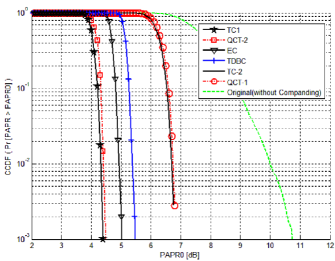Figure 3. PAPR performance comparision of original and companded signal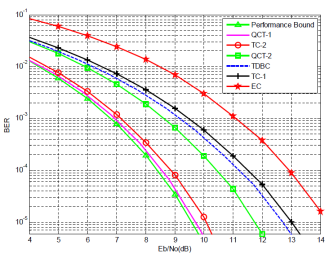Figure 4. BER performance comparison of various companding schemes

## CONCLUSION

The QCT provides extra degrees of freedom to design the companding function and hence by choosing the suitable values of design parameters of the proposed companding function, a good trade-off between the PAPR reduction and the BER can be achieved. The proposed QCT provides better PAPR reduction and BER performance in comparison to TC, EC and TDBC. QCT can achieve a minimum PAPR of 0dB, whereas TC and EC can achieve a minimum PAPR of 3dB and 4.771dB respectively. QCT-2 has superior PAPR performance in comparison to QCT-1 but its BER performance is inferior in comparison to QCT-1.

## References

1. L. J. Cimini, “Analysis and simulation of a digital mobile channel using orthogonal frequency division multiplexing,” IEEE Trans. Comm., vol. 33, no. 7, pp. 665–675, July 1985.
2. J. Bingham, “Multicarrier modulation for data transmission: an idea whose time has come,” IEEE Commun. Mag., vol. 28, no. 5, pp. 5–14, May 1990.
3. M. Schwartz, Mobile Wireless Communications. Cambridge University Press, 2005.
4. V. Nee, G. Awater, M. Morikura, H. Takanashi, M. Webster, and K. W. Halford, “New high-rate wireless LAN standards,” IEEE Commun. Mag., vol. 37, no. 12, pp. 82–88, December 1999.
5. I. Koffman and V. Roman, “Broadband wireless access solution based on OFDM access in IEEE 802.16,” IEEE Commun. Mag., vol. 40, no. 4, pp. 96–103, April 2002.
6. Van Nee R., Prasad R., OFDM for wireless Multimedia Communications, Artech House, 2003.
7. Weinstein S. B., Ebert P. M., “Data Transmission for Frequency-Division Multiplexing Using the Discrete Fourier Transform,” IEEE Transactions on Commun. Tech., vol. 19,no. 5, pp. 628–34, Oct. 1971
8. Chang R. W., “Synthesis of band-limited orthogonal signals for multichannel datatransmission”, Bell Systems Technical Journal, vol. 46, pp. 1775-1796, Dec. 1966.
9. Despain A. M., “Very Fast Fourier Transform Algorithms Hardware for Implementation”, IEEE Trans. Comp., Vol. C-28, no. 5, pp. 333-341, May 1979.
10. Bidet E., Castelain D., Joanblanq C., Senn P., “A fast single-chip implementation of 8192 complex point FFT”, IEEE Journal of Solid State Circ., Vol. 30, no. 3, pp. 300-305,Mar. 1995.
11. Chow P.S., Tu J.C. and Cioffi J.M., “Performance Evaluation of a Multichannel Transceiver System for ADSL and VHDSL services”, IEEE Journal on Selected Areas in Comm., vol. 9,no. 6, Aug. 1991.
12. R. V. Nee and A. D. Wild, “Reducing the peak-to-average power ratio of OFDM,” in Proc. IEEE Vehicular Technology Conference (VTC), vol. 3, New York, NY, USA, 1998, pp. 2072–2076.
13. Tao Jiang and Yiyan Wu, “An Overview: Peak-to-average power ratio reduction techniques for OFDM signals”, IEEE Transactions on Broadcasting, vol. 54, no. 2, pp. 257–268, June 2008.
14. O’Neill R., Lopes L. B., “Envelope variations and spectral splatter in clipped multicarrier signals,” in Proc. IEEE PIMRC’95, Toronto, Canada, pp. 71–75, Sept. 1995.
15. Li X., Cimini Jr. L.J., “Effect of Clipping and Filtering on Performance of OFDM”, IEEE Comm. Letters, vol. 2, no. 5, pp.131-133, July 1998.
16. Armstrong J., “Peak-to-average power reduction for OFDM by repeated clipping and frequencydomain filtering”, Electronics Letters, vol. 38, no. 5, pp. 246–247, Feb. 2002.
17. Chen H., Haimmovich A. M., “Iterative estimation and cancellation of clipping noise for OFDM signals,” IEEE Comm. Letters, vol. 7, no. 5, pp.246-247, July 2003.
18. Jiang T., Xiang W., Richardson P. C., Qu D., Zhu G., “On the Nonlinear Companding Transform for Reduction in PAPR of MCM Signals”, IEEE Transactions on Wireless Communications, vol. 6, no. 6, pp.2017-2021, June 2007.
19. Jiang T., Yang Y., Song Y., “Exponential companding transform for PAPR reduction in OFDM systems”, IEEE Transactions on Broadcasting, vol. 51, no. 2, pp. 244–248, June 2005.
20. Huang X., Lu J. H., Zheng J. L., Letaief K. B., Gu J., “Companding transform for reduction inpeak-to-average power ratio of OFDM signals”, IEEE Transactions on Wireless Comm., vol. 3, no. 6, pp. 2030–2039, Nov. 2004.
21. Aburakhia S. A., Badran E. F., Mohamed D. A. E., “Linear Companding Transform for the Reduction of Peak-to-Average Power Ratio of OFDM Signals”, IEEE Transactions on Broadcasting, vol. 55, no. 1, pp. 195-200, March 2009.
22. Hou J., Ge J. H., Li J., “Trapezoidal companding scheme for peak-to-average power ratio reduction of OFDM signals”, Electronics Letters, vol. 45, no. 25, pp. 1349-1351, Dec. 2009.
23. Jeng S. S., Chen J. M., “Efficient PAPR reduction in OFDM system based on a companding techniques with trapezium distribution”, IEEE Transactions on Broadcasting, vol. 57, no. 2, pp. 291-298, June 2011.
24. T. Hwang, C. Yang, G. Wu, S. Li, and G. Y. Lee, “OFDM and its wireless application: A survey,” IEEE Trans. Veh. Technol., vol. 58, no. 4, pp. 1673–1694, May 2009.
25. Y. Wang and B. Ai, “PAPR reduction in OFDM systems via nonlinear companding transform”, in 8th International Conference on Wireless Communications, Networking and Mobile Computing (WiCOM), pp. 172-175, Sept. 2012.

#### Cite This Work

To export a reference to this article please select a referencing stye below:

## Related Services

View all

### DMCA / Removal Request

If you are the original writer of this essay and no longer wish to have the essay published on the UK Essays website then please:Prices from
###### £28

Undergraduate 2:2 • 250 words • 7 day delivery

Delivered on-time or your money back

##### Rated 4.6 out of 5 by(146 Reviews)